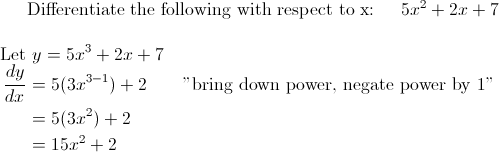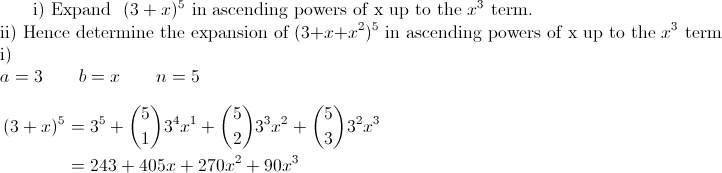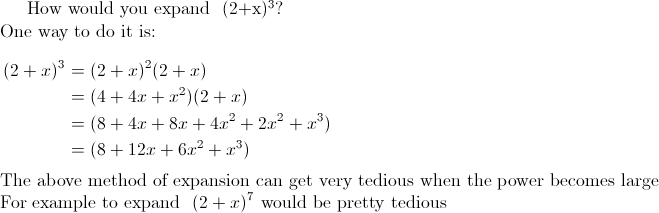## Posts

Showing posts from May, 2010

### IndicesIndices is closely related to Surds and Logarithms What's Next Click here to check out how to solve log equations Click here to check out how to rationalize surds Click here for Contents Page

### Applications of Differentiation Part II

Tangents and Normal Example 4 The point A lies on the curve $y = x^2 -x + c$ where c is a constant. The tangent to the curve at A is $y = 3x - 1$. Find the equation of the normal to the curve at A. Recall equation of straight line: $y = mx +c \qquad \text{m is gradient}$ Since tangent to the the curve at A is $y = 3x + 5$ , gradient of tangent is 3 We can calculate the gradient using $\frac{dy}{dx}$ $\frac{dy}{dx} = 2x -1$ To obtain x coordinates of A, we set $\frac{dy}{dx} = 3$ $2x -1 = 3 \qquad x = 2$ To determine the equation of normal we need to know the gradient of the normal and a point on the normal Gradient of the normal is the negative reciprocal of gradient of tangent Gradient of normal = $-\frac{1}{3}$ A is a point on the tangent as well as the normal When $x = 2 \qquad y = 3(2)-1 = 5$ Sub $x= 2 \quad y = 5 \quad m=-\frac{1}{3}$ into $y = mx +c$ \begin{aligned} 5 &= -\frac{1}{3}(2) + c \\ c &= 5\frac{2}{3} \\ \end{aligned} Equation

### Applications of Differentiation Part I

Tangents and Normals Equation of a line is given by: $y = mx + c$ where m is the gradient and c is the y intercept The gradient at a particular point on a curve can be found using $\frac{dy}{dx}$ To find the equation of a line, you will need a point on the line and the gradient of the line Example 1 The equation of a curve is $y = \frac{5}{1 + x^2}$ Find the equation of the tangent to the curve at the point where x = 2 . First we differentiate y wrt x To make our job easier, we rewrite y as $y = 5( 1 + x^2)^{-1}$ \begin{aligned} \frac{dy}{dx} &= 5(-1)(1+x^2)^{-1-1}(2x) \qquad \text{applying chain rule} \\ &= (-10x)(1+x^2)^{-2} \\ &= \frac{-10x}{(1+x^2)^{2}}\\ \end{aligned} In order to find the equation of the tangent, we need to determine the gradient of the tangent. When x = 2 , $\frac{dy}{dx} = \frac{-10(2)}{1+2^2}} = -4$ Now that we have determined the gradient, we need to find a point on the tangent The point on the tangent is also the point at w

### Differentiation Part IIProduct rule Example 1 Example 2 Quotient rule Example 3 Example 4 What's Next? Now that you learnt the techniques of differentiation, it time to apply them click here for Applications of Differentiation Part I: Tangents and Normals click here for Applications of Differentiation Part III: Stationary Values click here for Applications of Differentiation Part IV: Connected Rates of Change

### Differentiation Part IBasic Differentiation Differentiating term by term Example 1 Example 2 Example 3 Chain Rule Example 4 Example 5 What's Next? Click here for more techniques of differentiation: Product Rule and Quotient Rule Click here for Contents Page

### Binomial Theorem Part IIExample 1 ii) Example 2 Example 3

### Binomial Theorem Part IIntroduction Another way is to use the Binomial Theorem Example 1 Example 2 The (r+1)th term The (r+1)th term is given in the formula list: $\ {n \choose r}a^{n-r}b^r$ Example 3   Example 4 Hence the term independent of x is 15